UPSC  >  Important Concepts: HCF & LCM

# Important Concepts: HCF & LCM - CSAT Preparation - UPSC

## HCF: Highest Common Factor

• Every number has some factors, but if two or more numbers taken together can have one or more common factors. Out of those common factors, the greatest among them will be the highest common divisor or highest common factor of those numbers.
• Such as 12 and 18 have 1, 2, 3 and 6 as common factors, but among them, 6 is the highest common factor. So H.C.F. of 12 and 18 is 6.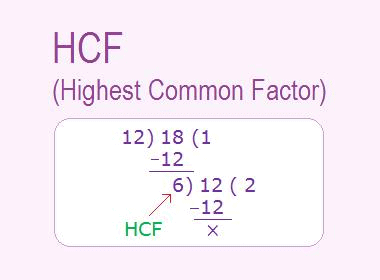### ➢ Methods of Finding H.C.F

(a) Factorisation Method

• Express each number as the product of primes and take the product of the least powers of common factors to get the H.C.F.

(b) Division Method

• Divide the larger number by the smaller one. Now divide the divisor by the remainder. Repeat the process of dividing the preceding divisor by the remainder last obtained, till the remainder zero is obtained.
• The last divisor is the required H.C.F.

## LCM: Least Common Multiple

• When we write the multiples of any two or more numbers taken together, we find that they have some common multiples. Out of those common multiples, the smallest among them will be the least common multiple of those numbers.
• Such as 12 and 18 have 36, 72, 108, 144….. as common multiples, but 36 is the least among them. So, L.C.M. of 12 and 18 is 36.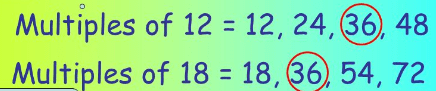### ➢ Methods of Finding L.C.M

(a) Factorisation Method

• Resolve each one of the given numbers into prime factors. Then the product of the highest power of all the factors gives the L.C.M.

Example 1: Find the L.C.M of 2, 4, 6, 8, 10.

• Write the numbers as the product of primes.
• 2, 22, 2× 3, 23, 2× 5
• Take the highest powers of all the primes. i.e., 23 × 3 × 5 = 120.

Example 2: Find the H.C.F. of 2, 4, 6, 8, 10 using the factorization method.

• For H.C.F, take the common prime factors. i.e., 2. (This is only the prime factor common in all the numbers).

### ➢ Important Concepts

(i) Product of two numbers = L.C.M. × H.C.F.

(ii) Product of n numbers = L.C.M of n numbers × Product of the HCF of each possible pair.

Or

If the HCF of all the possible pairs taken is same then we will have:

Product of n numbers = L.C.M of n numbers × (H.C.F of each pair)(n – 1)

(iii) If the ratio of numbers is a : b and H is the HCF of the numbers. Then,
LCM of the numbers = H × a × b = HCF × Product of the ratios.

(vi) H.C.F of fractions =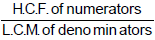(vii) L.C.M. of fractions =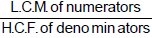(viii) If HCF (a, b) = H1 and HCF (c, d) = H2, then HCF (a, b, c, d) = HCF (H1, H2).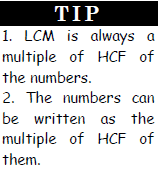### ➢ Important Results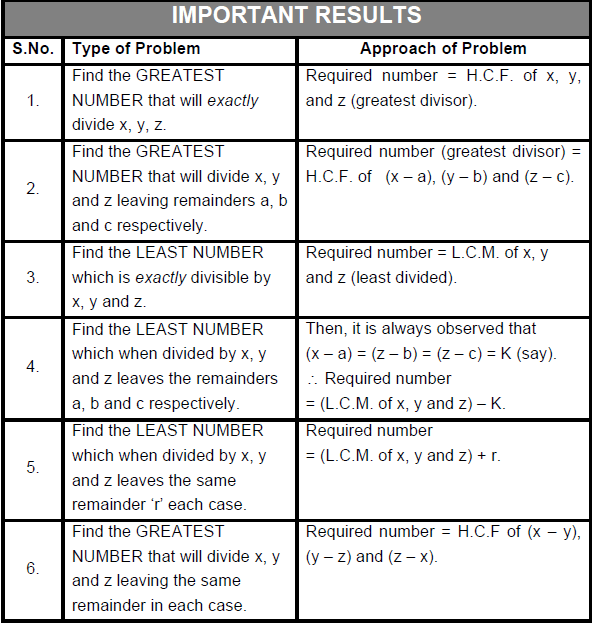Example 3: What is the greatest number which exactly divides 110, 154 and 242?

• The required number is the HCF of 110, 154 & 242.
• 110 = 2 × 5 × 11
• 154 = 2 × 7 × 11
• 242 = 2 × 11 × 11
• ∴ HCF = 2 × 11 = 22

Example 4: What is the greatest number, which when divides 3 consecutive odd numbers produces a remainder of 1.

• If x, y, z be 3 consecutive odd numbers, then the required number will be the HCF of x – 1, y – 1 and z – 1.
• Since x-1, y-1 & z-1 are 3 consecutive even integers, their HCF will be 2. So the answer is 2.

Example 5: What is the highest 3 digit number, which is exactly divisible by 3, 5, 6 and 7?

• The least no. which is exactly divisible by 3, 5, 6, & 7 is LCM (3, 5, 6, 7) = 210. So, all the multiples of 210 will be exactly divisible by 3, 5, 6 and 7.
• So, such greatest 3 digit number is 840 = (210 × 4).

Example 6: In a farewell party, some students are giving pose for photographs, if the students stand at 4 students per row, 2 students will be left if they stand 5 per row, 3 will be left and if they stand 6 per row 4 will be left. If the total number of students are greater than 100 and less than 150, how many students are there?

• If ‘N’ is the number of students, it is clear from the question that if N is divided by 4, 5, and 6, it produces a remainder of 2, 3, & 4 respectively.
• Since (4 – 2) = (5 – 3) = (6 – 4) = 2, the least possible value of N is LCM (4, 5, 6) – 2 = 60 – 2, = 58.
• But, 100 < N < 150. So, the next possible value is 58 + 60 = 118.

Example 7: There are some students in the class. Mr X brought 130 chocolates and distributed to the students equally; then he was left with some chocolates. Mr Y brought 170 chocolates and distributed equally to the students. He was also left with the same no of chocolates as MrX was left. Mr Z brought 250 chocolates, did the same thing and left with the same no of chocolates. What is the max possible no of students that were in the class?

• The question can be stated as, what is the highest number, which divides 130, 170 and 250 gives the same remainder, i.e. HCF ((170 −130),(250 −170),(250 −130)).
i.e. HCF (40, 80, 120) = 40.

Question for Important Concepts: HCF & LCM
Try yourself:Number of students who have opted for subjects A, B and C are 60, 84 and 108 respectively. The examination is to be conducted for these students such that only the students of the same subject are allowed in one room. Also, the number of students in each room must be the same. What is the minimum number of rooms that should be arranged to meet all these conditions?

Question for Important Concepts: HCF & LCM
Try yourself:A red light flashes three times per minute and a green light flashes five times in 2 min at regular intervals. If both lights start flashing at the same time, how many times do they flash together in each hour?

Question for Important Concepts: HCF & LCM
Try yourself:A is the set of positive integers such that when divided by 2, 3, 4, 5, 6 leaves the remainders 1, 2, 3, 4, 5, respectively. How many integers between 0 and 100 belong to set A?

Question for Important Concepts: HCF & LCM
Try yourself:In Sivakasi, each boy’s quota of match sticks to fill into boxes is not more than 200 per session. If he reduces the number of sticks per box by 25, he can fill 3 more boxes with the total number of sticks assigned to him. Which of the following is the possible number of sticks assigned to each boy?

Question for Important Concepts: HCF & LCM
Try yourself:A new flag is to be designed with six vertical stripes using some or all of the colours yellow, green, blue and red. Then, the number of ways this can be done such that no two adjacent stripes have the same colour is

The document Important Concepts: HCF & LCM | CSAT Preparation - UPSC is a part of the UPSC Course CSAT Preparation.
All you need of UPSC at this link: UPSC

## CSAT Preparation

197 videos|151 docs|200 tests

## FAQs on Important Concepts: HCF & LCM - CSAT Preparation - UPSC

 1. What is HCF and LCM?Ans. HCF stands for Highest Common Factor and LCM stands for Least Common Multiple. HCF is the greatest number that divides two or more numbers exactly. LCM is the smallest number that is a multiple of two or more numbers.
 2. How to find HCF and LCM of two or more numbers?Ans. To find HCF, we need to find the common factors of the given numbers and select the greatest among them. To find LCM, we need to find the multiples of the given numbers and select the smallest among them.
 3. What is the relationship between HCF and LCM?Ans. The product of two numbers is equal to the product of their HCF and LCM. That is, if a and b are two numbers, then a x b = HCF(a,b) x LCM(a,b).
 4. Can HCF of two numbers be greater than their LCM?Ans. No, HCF of two numbers cannot be greater than their LCM. This is because HCF is a factor of LCM. Hence, HCF is always less than or equal to LCM.
 5. What is the difference between HCF and GCD?Ans. HCF and GCD (Greatest Common Divisor) are the same. They both represent the greatest number that divides two or more numbers exactly. However, HCF is commonly used in India, while GCD is commonly used in Western countries.

## CSAT Preparation

197 videos|151 docs|200 testsExplore Courses for UPSC exam### How to Prepare for UPSC

Read our guide to prepare for UPSC which is created by Toppers & the best Teachers
Signup to see your scores go up within 7 days! Learn & Practice with 1000+ FREE Notes, Videos & Tests.
10M+ students study on EduRev
Track your progress, build streaks, highlight & save important lessons and more!
Related Searches

,

,

,

,

,

,

,

,

,

,

,

,

,

,

,

,

,

,

,

,

,

;